## 初阶数据结构——二叉树题目

chatgpt/2023/10/4 8:55:17

• 一、单值二叉树
• 二、检查两颗树是否相同
• 三、另一棵树的子树
• 四、二叉树的前序遍历
• 五、对称二叉树

# 一、单值二叉树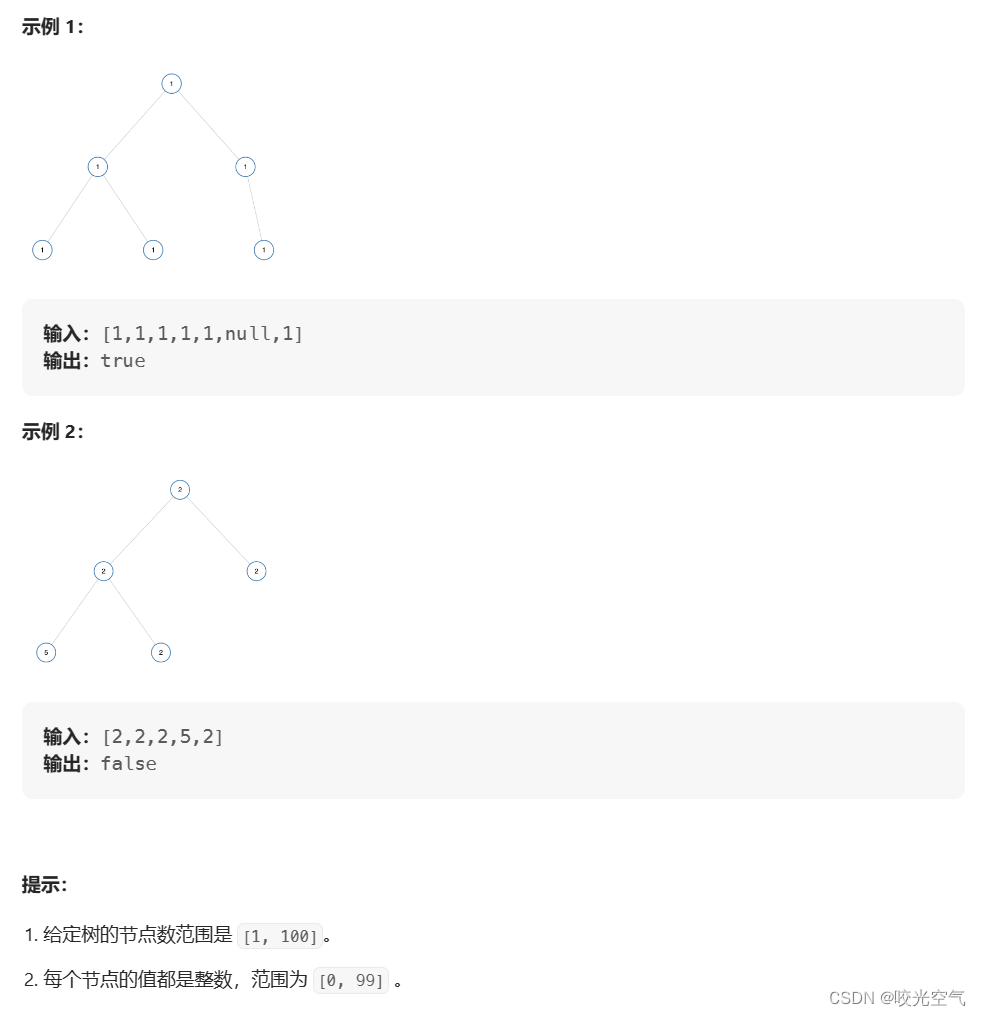``````if(root->val != root->right->val)
``````

``````return isUnivalTree(root->left) && isUnivalTree(root->right);
``````

``````bool isUnivalTree(struct TreeNode* root)
{if(!root) return true;if(root->left && root->val != root->left->val)return false;if(root->right && root->val != root->right->val)return false;return isUnivalTree(root->left) && isUnivalTree(root->right);
}
``````

# 二、检查两颗树是否相同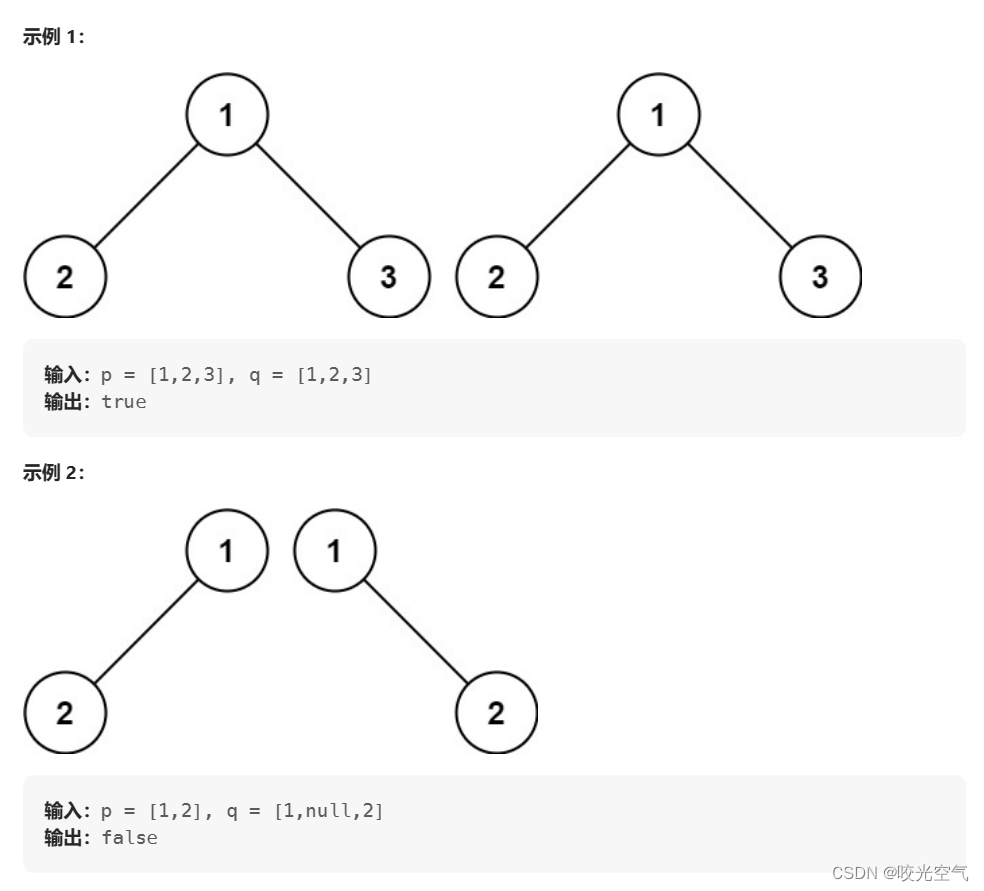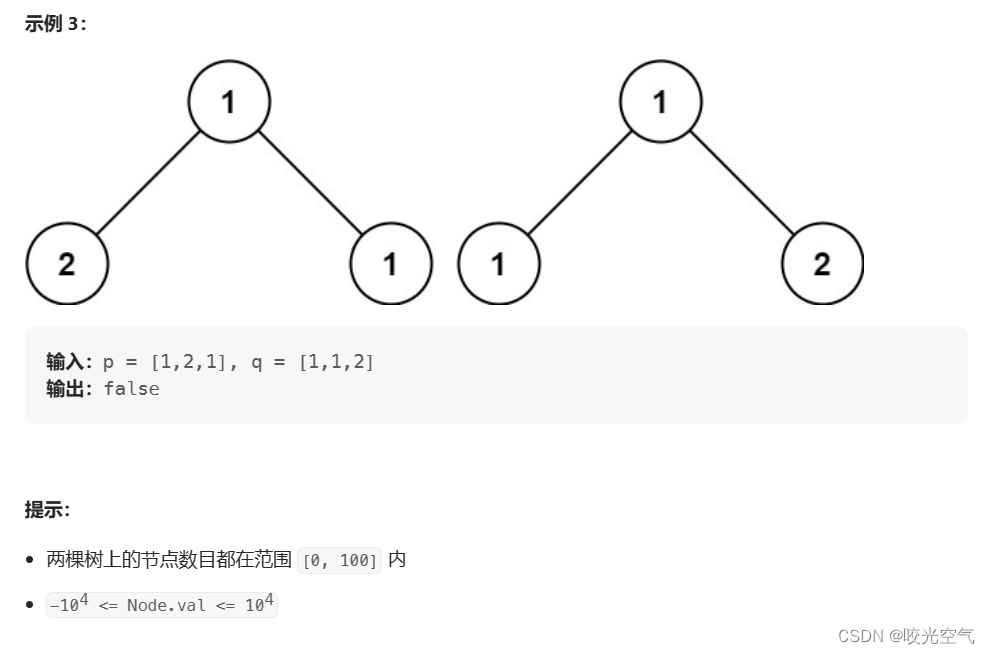``````bool isSameTree(struct TreeNode* p, struct TreeNode* q){if(p == NULL && q == NULL) return true;if(p == NULL || q == NULL) return false;if (p->val != q->val) return false;return isSameTree(p->left, q->left) && isSameTree(p->right, q->right);
}
``````

# 三、另一棵树的子树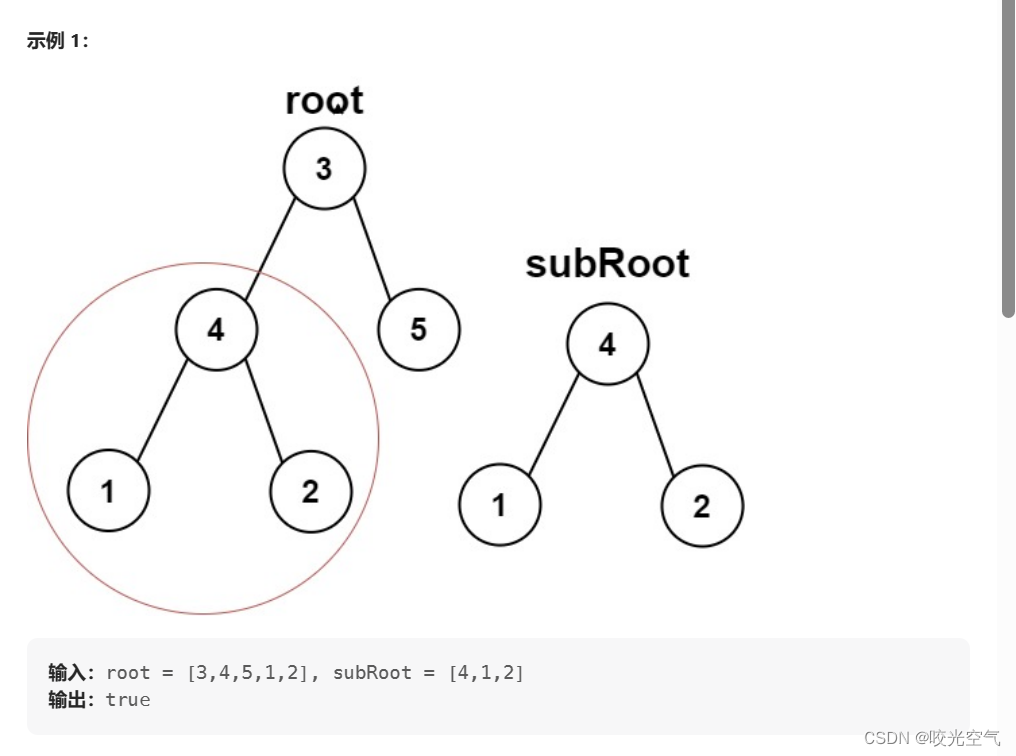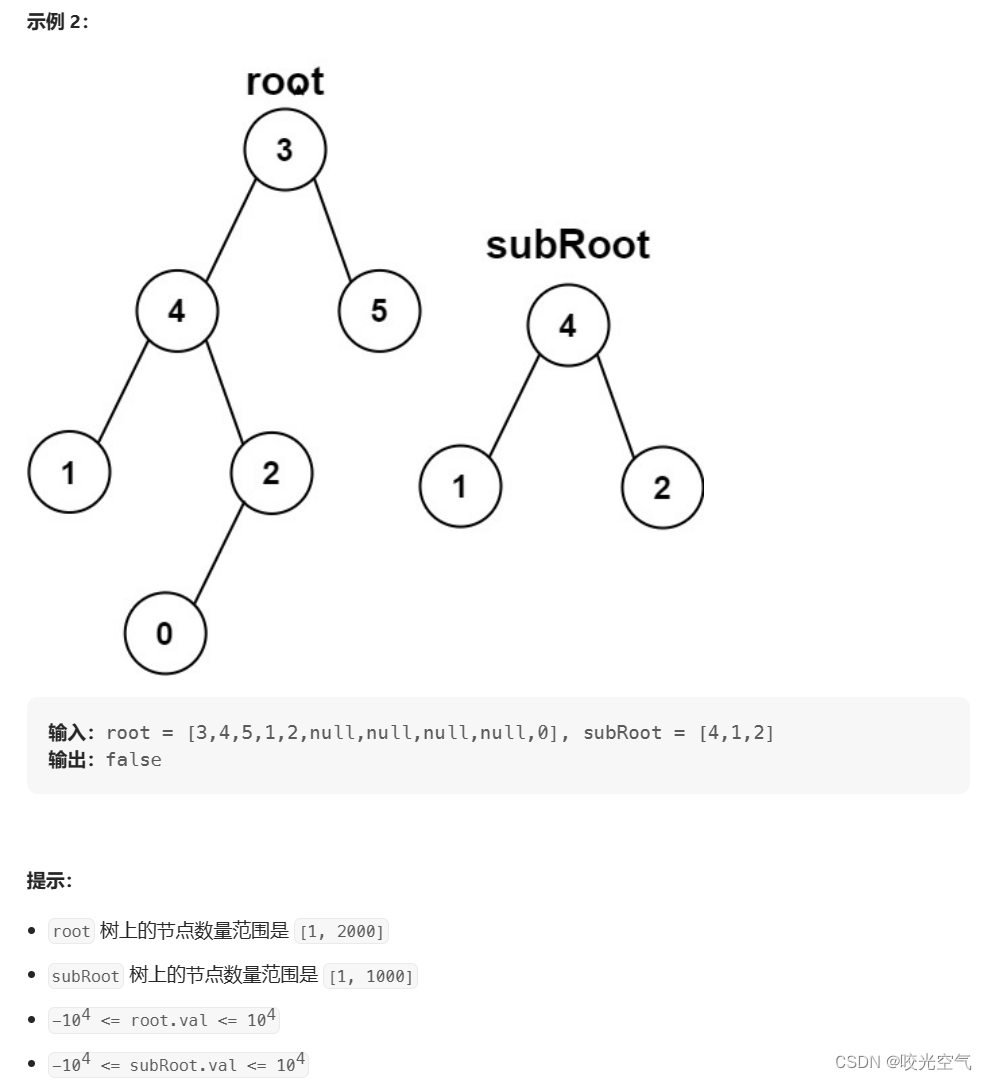``````bool isSameTree(struct TreeNode* p, struct TreeNode* q) {if(p == NULL && q == NULL) return true;if(p == NULL || q == NULL) return false;if(p->val != q->val) return false;return isSameTree(p->left, q->left) && isSameTree(p->right, q->right);
}bool isSubtree(struct TreeNode* root, struct TreeNode* subRoot) {if(!root) return false;if(isSameTree(root, subRoot)) return true;return isSubtree(root->left, subRoot) || isSubtree(root->right, subRoot);
}
``````

# 四、二叉树的前序遍历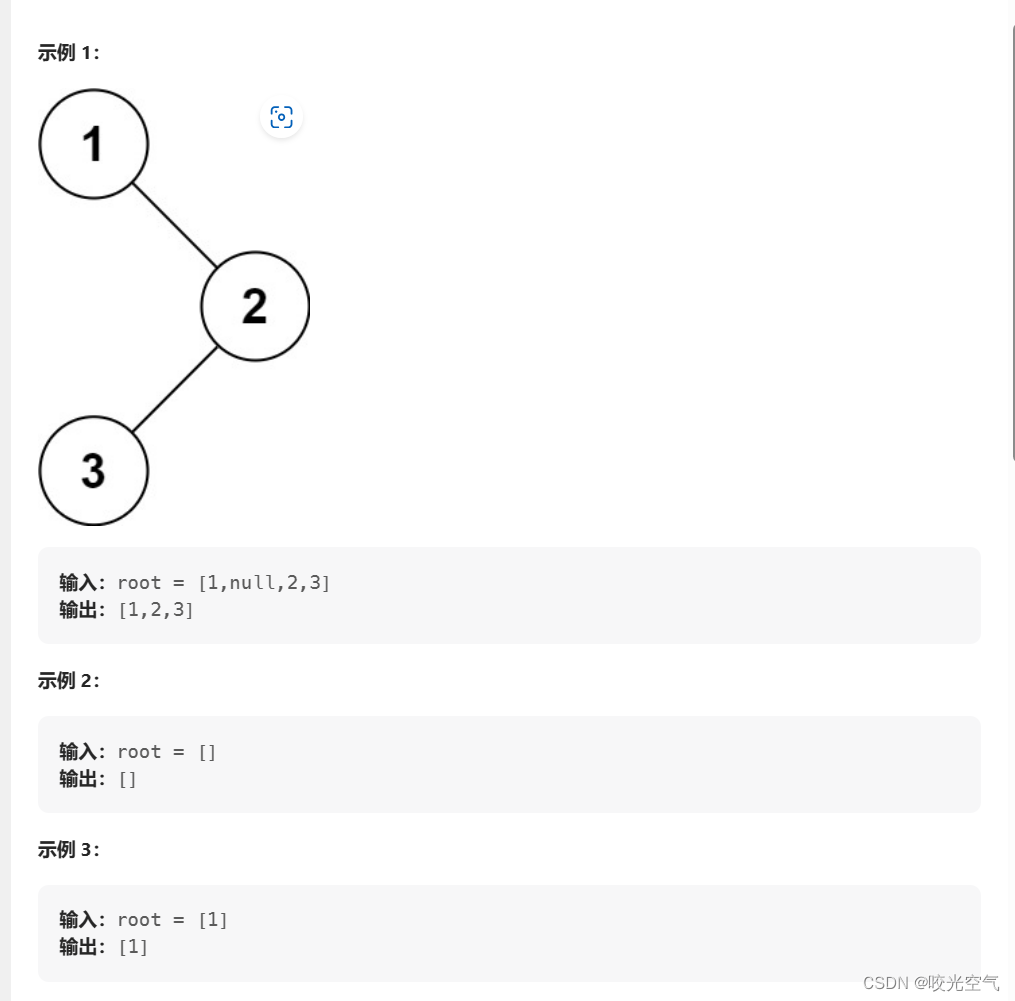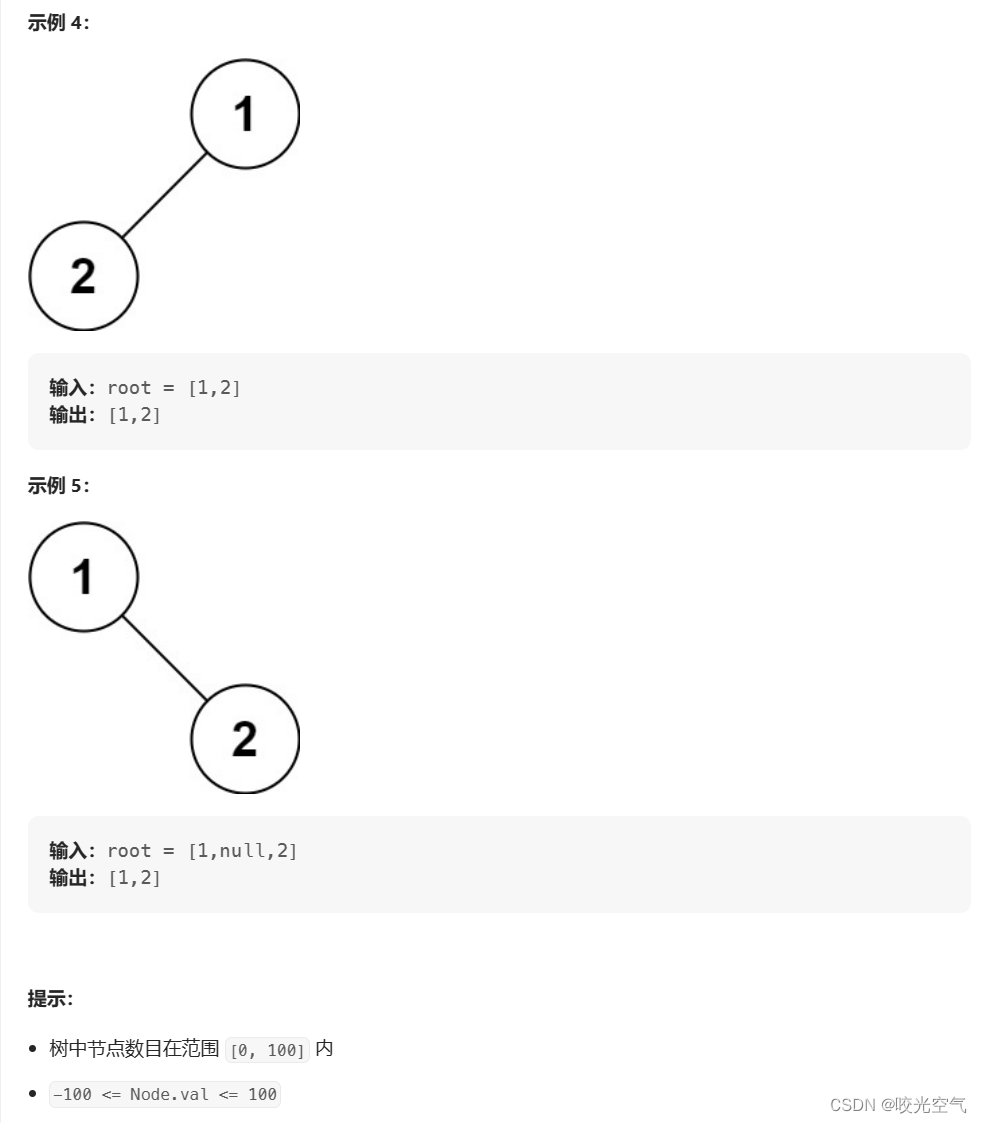``````int TreeSize(struct TreeNode* root)
{if root == NULL ? 0 : TreeSize(root->left) + TreeSize(root->right) + 1;
}void preorder(struct TreeNode* root, int* array, int* i)
{if(root == NULL){return;}array[(*i)++] = root->val;preorder(root->left, array, i);preorder(root->right, array, i);
}int* preorderTraversal(struct TreeNode* root, int* returnSize){*returnSize = TreeSize(root);int* array = (int*)malloc(sizeof(int) * (*returnSize));int i  = 0;preorder(root, array, &i);return array;
}
``````

# 五、对称二叉树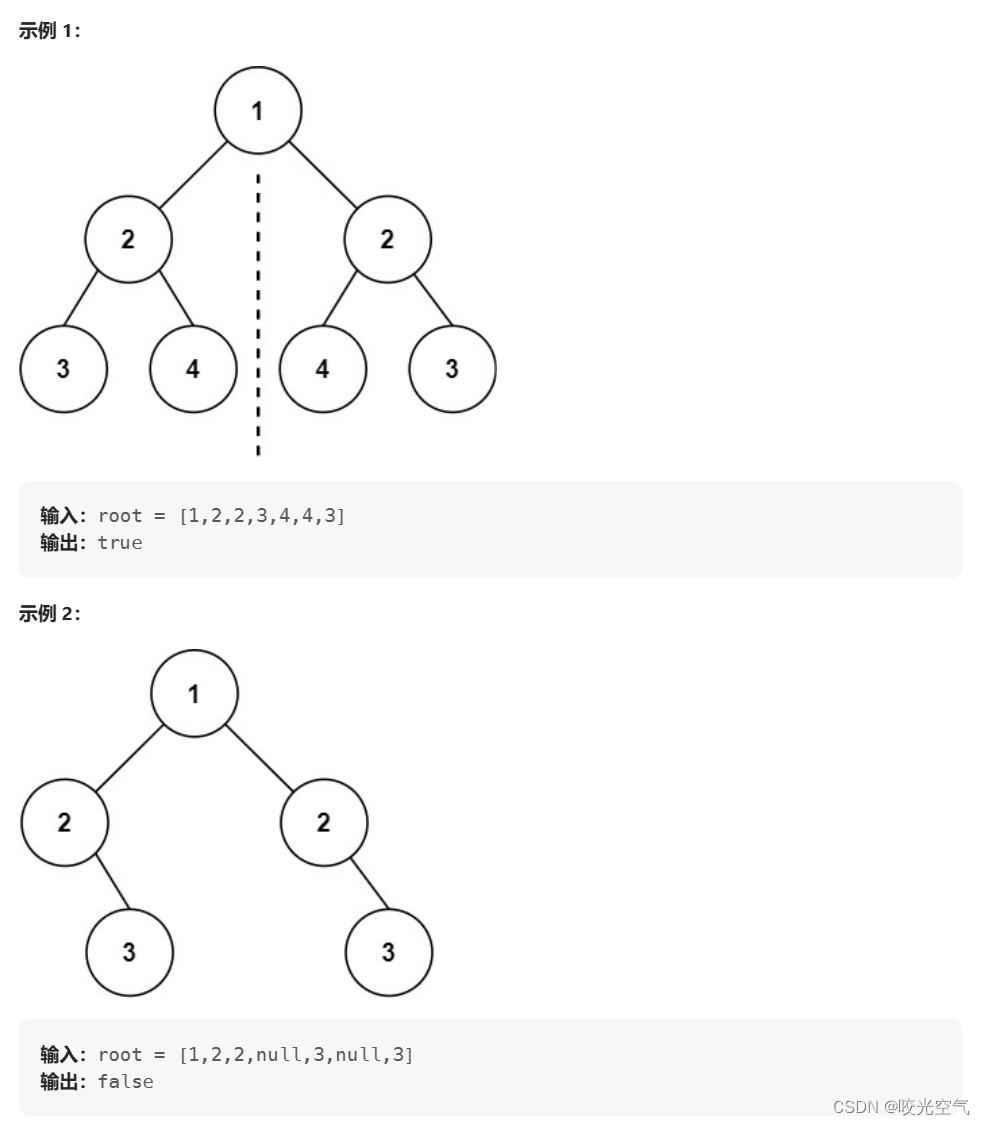``````bool isSameTree(struct TreeNode* p, struct TreeNode* q){if(!p && !q){return true;}if((p == NULL || q == NULL) || (p->val != q->val)){return false;}return isSameTree(p->left, q->right) && isSameTree(p->right, q->left);
}bool isSymmetric(struct TreeNode* root){if(root == NULL){return true;}return isSameTree(root->left, root->right);
}
``````